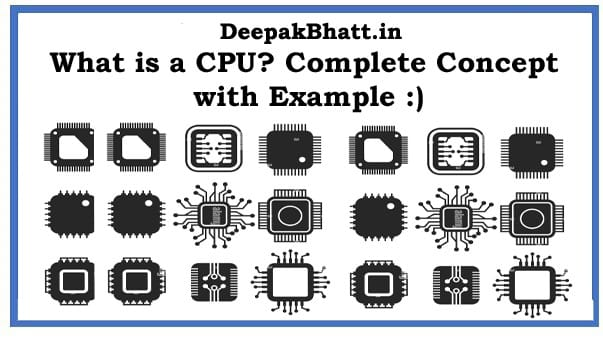71 / 100

What is a CPU: The full form is the Central Processing Unit. With its help, instructions and directions are accessed on the computer.

And it serves to direct the accompanying activity such as the input-output process. It is also called a processor. For example, if we do calculations on a computer. And input some data there.

So by doing the calculation inside the direct CPU, it shows 4 outputs except 2 + 2.

While giving instructions to the CPU, we easily control the input by arithmetic means, and calculations are done with the CPU using the control unit and arithmetic logic unit.

Different units inside the CPU work to perform calculations. Where units like control units, arithmetic logic units, etc. perform the task of performing calculations.

## What is a CPU?

The full form is Central Processing Unit. This is a small chip. What is installed in the computer is the backbone of the computer.

The computer cannot be run without its help. Arithmetic like logic unique etc. has been used inside it. It is also called a processor.

Because it calculates the input. and converts the output.

### Features

It has the following features which are mentioned below.

• The CPU gives instructions to the computer.
• It is the brain of the computer.
• Operates all the parts of the computer.
• It imports and exports data

### Component

Now we will talk about the CPU structure. What is the structure of the CPU? We need to understand this.

That’s how the CPU is made. And what components were used in it? And what components work inside the CPU. The information of all of them comes in the CPU structure.

To understand CPU structure, we have to know about the Components of the CPU. Inside it comes Control Unit, Logical Unit, Arithmetic Unit.

There are various components of the CPU. Like Memory Unit, Arithmetic Logical Unit, Control Unit, all these units are explained in detail below.

#### Memory Unit

The main component of the CPU is the memory unit. A memory unit is a storage unit. which stores the data. It helps in exchanging data. We call this unit as Internal Storage Unit Primary Storage Main Memory RAM. One primary memory the other secondary memory.

##### Memory Unit Functions
• The memory unit stores the instruction.
• Stores those instructions before going to the output device.
• Leaves all the input-output devices with the main memory.

#### Control Unit

Controls all the parts of the computer. All the parts of a computer when instructions are given to them. So don’t make any mistake while giving instructions. Therefore, controls them and helps to see the output by delivering the instructions in the right way.

##### Control Unit Functions
• Controls all the units of the computer.
• Coordinates all the units that work in taking and giving instructions.
• Provides instruction of memory.
• Communicates with Input-Output with Instruction

#### Arithmetic Logic Unit

There are two units inside the Arithmetic Logic Unit. In which there are arithmetic units and logic units. We will discuss these two units.

• Arithmetic Unit.
• Logic Unit.

Arithmetic Unit

The arithmetic unit computer controls mathematical problems. and solves them. Arithmetic instruction includes addition, subtraction, multiplication, division. It solves these complex problems easily.

Logic Unit

The logic unit is different from the arithmetic unit. In this, Greater, Less Equal, etc. are used. And the work of solving them is done by the logic unit.

### Frequently Asked Questions

#### What is CPU Bench Mark?

Its’s benchmark is a result of the testing and testing of the computer or CPU. It serves to measure and compare all the components of the CPU.

What is the temperature of the best CPU and as many CPUs use this method to compare and along with it some features are also included in it. Like clock speed, the number of instructions, etc.

#### What does the CPU work for?

The Complete form of CPU is Central Processing Unit. CPU is a system that acts as the brain of the computer. This is called a processor. And with this, it is also called a microprocessor. It serves to give instruction to the program. And keeps full responsibility for the computer.

#### State four functions

• Provides CPU Instruction
• Is the brain of the computer
• Brings program instruction to memory
• Controls the input output

#### Name the five parts of CPU?

The 5 parts of the CPU are as follows. Below you can see 5 parts at the base of the complete list.

• Register unit
• Arithmetic Logic Unit
• Control unit
• Random Access Memory
• Buses

#### What happens inside the CPU chip?

Different layers are made inside the CPU. Which is prepared from physical equipment. Inside it, there is a combination of transistor and logic circuit gate, etc.

### Conclusion

Finally, friends, I gave you information about the CPU, you must have understood the concept of CPU.

Along with this, you got information about the features and components of the CPU, with this if you like this post.

So do share with your friends. And bookmark our website for upcoming new posts. And also subscribe so that you get regular notifications.

5/5 - (1 vote)